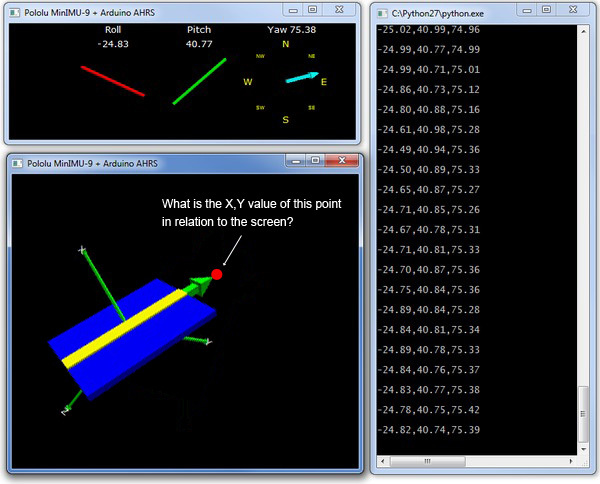# MinIMU-9 (AHRS) Getting X,Y point?

Hey guys,
I need some help from the math wizards hereI was wondering if there is a way to determine the X,Y value of this point (The red dot) in this image --attached.
I’d like to get 2D (x,y) coordinate of where the green arrow is pointing in the AHRS…

I really appreciate the help!

C.Hello, C.

What do you mean by “in relation to the screen”? In general, you can use these formulas to convert roll, pitch, and yaw to x, y, and z:

x = cos(yaw)*cos(pitch)
y = sin(yaw)*cos(pitch)
z = sin(pitch)

- Jeremy

Hi Jeremy,
Thanks for your quick reply, I should’ve explained a little more…Ok, so in relation to the screen I mean the resolution of the user’s monitor. For the time being I will hard-code this as 1680pixels wide x 1050 pixels high
What I would like to do is is divide that resolution into a grid where 0,0 is the center of the monitor, and the X,Y becomes that point so that I can map the mouse to it.

I’m pretty sure I already tried those calculations you mentioned but it was 3am, so let me try again, haha.

Thanks again,